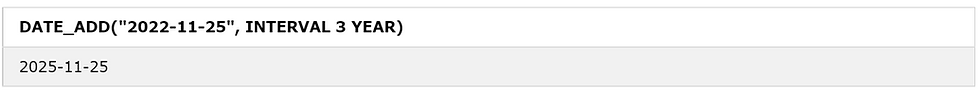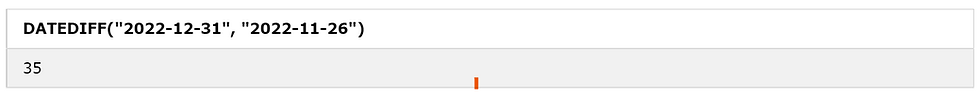top of pageSearch

# MYSQL:: Date FunctionsSQL stands for structured Query Language. SQL is a computer language for storing, manipulating, and retrieving data in a relational database. In other words, we can interact with the database with SQL Queries.

MYSQL Date Functions:

MYSQL date functions allow you to manipulate date and time data effectively. You can use the date functions for various operations such as adding days to a date, calculating the difference between two dates, or getting parts of a date.

• CURRENT_DATE

• SYSDATE

• CURRENT_TIME

• CURRENT_TIMESTAMP

• DATEDIFF

• LAST_DAY()

• DATE_FORMAT

• EXTRACT

EXTRACT YEAR FROM DATE

EXTRACT MONTH FROM DATE

EXTRACT DAY FROM DATE

CURRENT_DATE:

The CURRENT_DATE() function returns the current date.

The following statement returns the current date:

SELECT CURRENT_DATE();

Here is the output:SYSDATE:

The SYSDATE() function returns the current date.

The following statement returns the current date:

SELECT SYSDATE();

Here is the output:CURRENT_DATE AND SYS_DATE both return the current date.CURRENT_DATE is a function and SYSDATE is not.

 SYSDATE CURRENT_DATE SYSDATE is the current date on the server where the database resides. CURRENT_DATE is the date of the client from where you are connecting.

CURRENT_TIME:

The CURRENT_TIME() function returns the current time.

The following statement returns the current time:

SELECT CURRENT_TIME();

Here is the output:CURRENT_TIMESTAMP:

The CURRENT_TIMESTAMP() function returns the current date and time.

The following statement returns the current date and time:

SELECT CURRENT_TIMESTAMP();

Here is the output:The DATE_ADD() function adds a time/date interval to a date and then returns the date.

The following statement returns the date after adding 10 days to the date:

Here is the output:The following statement returns the date after adding 1 month to the date:

Here is the output:The following statement returns the date after adding 3 years to the date:

Here is the output:DATEDIFF:

The DATE_DIFF() function returns the number of days between two date values.

The following statement returns the number of days between two date values:

SELECT DATEDIFF("2022-12-31", "2022-11-26");

Here is the output:LAST_DAY:

The LAST_DAY() Function returns the last day of the month.

The following statement returns the last day of the month.

SELECT LAST_DAY("2022-12-25");

Here is the output:DATE_FORMAT:

The DATE_FORMAT function formats a date as specified.

The following statement returns the week, month, day, and year format of the given date.

SELECT DATE_FORMAT("2022-11-25", "%W %M %e %Y");

Here is the output:Another example of DATE_FORMAT()

The following statement returns the month, day, and year format of the given date.

SELECT DATE_FORMAT("2022-11-25", "%M %d %Y");

Here is the output:EXTRACT:

The EXTRACT() function extracts a part from a given date.

EXTRACT YEAR FROM DATE:

The following statement extracts the YEAR from the given date.

SELECT EXTRACT(YEAR FROM "2022-11-25")

Here is the output:EXTRACT MONTH FROM DATE:

The following statement extracts the MONTH from the given date.

SELECT EXTRACT(MONTH FROM "2022-11-25");

Here is the output:EXTRACT DAY FROM DATE:

The following statement extracts the DAY from the given date.

SELECT EXTRACT(DAY FROM "2022-11-25");

Here is the output: